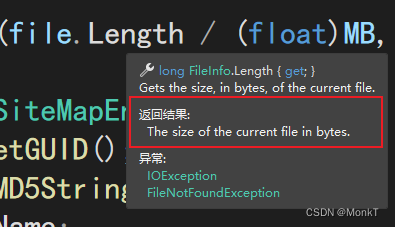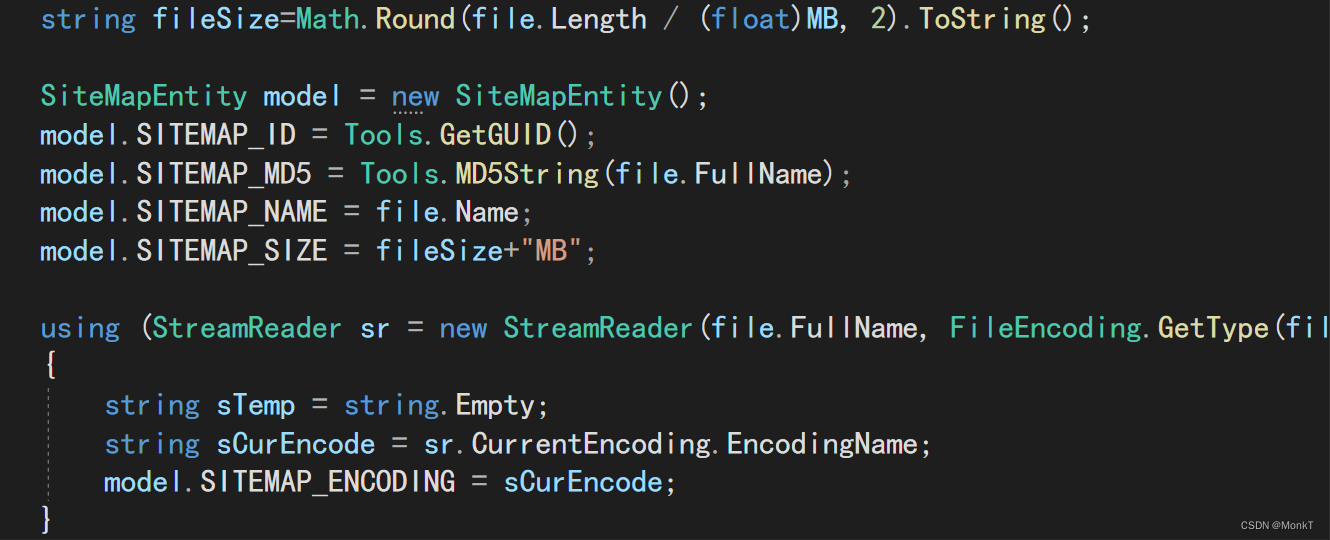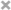# C# .Net Core bytes转换为GB/MB/KB 算法

c#本身提供的文件大小是bytes​编辑

`````` int GB = 1024 * 1024 * 1024;//定义GB的计算常量
int MB = 1024 * 1024;//定义MB的计算常量
int KB = 1024;//定义KB的计算常量
``````道理是一样的我这里的用MB举例

``string fileSize=Math.Round(file.Length / (float)MB, 2).ToString();``​编辑

c#本身提供的文件大小是bytes​编辑

`````` int GB = 1024 * 1024 * 1024;//定义GB的计算常量
int MB = 1024 * 1024;//定义MB的计算常量
int KB = 1024;//定义KB的计算常量
``````道理是一样的我这里的用MB举例

``string fileSize=Math.Round(file.Length / (float)MB, 2).ToString();``​编辑